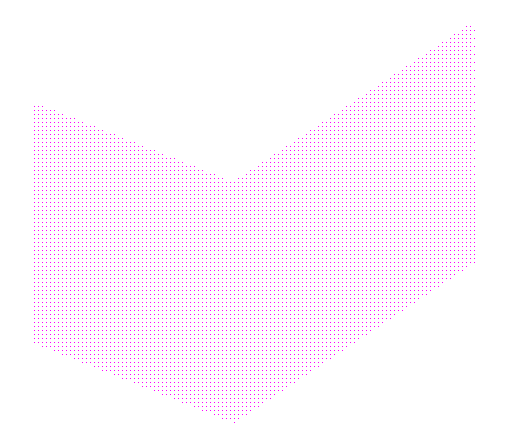2019-04-10 14:56

# 求助大佬 ，，，，如何将以下程序生成的图片转换成bmp格式。#include
#include

const int POINTNUM = 6;

typedef struct XET
{
float x;
float dx, ymax;
XET* next;
}AET, NET;

struct point
{
float x;
float y;
}

polypoint[POINTNUM] = { 20,30,70,10,130,50,130,110,70,70,20,90,};

void PolyScan()
{
int MaxY = 0;
int i;
for (i = 0; i < POINTNUM; i++)
{
if (polypoint[i].y > MaxY)
MaxY = polypoint[i].y;
}

``````AET *pAET = new AET;
pAET->next = NULL;

NET *pNET;
for (i = 0; i <= MaxY; i++)
{
pNET[i] = new NET;
pNET[i]->next = NULL;
}
glClear(GL_COLOR_BUFFER_BIT);
glColor3f(1, 0, 1);
glBegin(GL_POINTS);

for (i = 0; i < MaxY; i++)              //扫描建立NET表
{
for (int j = 0; j < POINTNUM; j++)
{
if (polypoint[j].y == i)
{
if (polypoint[(j - 1 + POINTNUM) % POINTNUM].y > polypoint[j].y)
{
NET *p = new NET;
p->x = polypoint[j].x;
p->ymax = polypoint[(j - 1 + POINTNUM) % POINTNUM].y;
p->dx = (polypoint[(j - 1 + POINTNUM) % POINTNUM].x - polypoint[j].x) / (polypoint[(j - 1 + POINTNUM) % POINTNUM].y - polypoint[j].y);
p->next = pNET[i]->next;
pNET[i]->next = p;
}
if (polypoint[(j + 1 + POINTNUM) % POINTNUM].y > polypoint[j].y)
{
NET *p = new NET;
p->x = polypoint[j].x;
p->ymax = polypoint[(j + 1 + POINTNUM) % POINTNUM].y;
p->dx = (polypoint[(j + 1 + POINTNUM) % POINTNUM].x - polypoint[j].x) / (polypoint[(j + 1 + POINTNUM) % POINTNUM].y - polypoint[j].y);
p->next = pNET[i]->next;
pNET[i]->next = p;
}
}
}
}
for (i = 0; i <= MaxY; i++)    //建立更新活性边表
{
NET *p = pAET->next;
while (p)
{
p->x = p->x + p->dx;
p = p->next;
}

AET *tq = pAET;             //AET按照X值从小到大排序
p = pAET->next;
tq->next = NULL;
while (p)
{
while (tq->next && p->x >= tq->next->x)
tq = tq->next;
NET *s = p->next;
p->next = tq->next;
tq->next = p;
p = s;
tq = pAET;
}

AET *q = pAET;              //从AET中删除ymax == i的结点
p = q->next;
while (p)
{
if (p->ymax == i)
{
q->next = p->next;
delete p;
p = q->next;
}
else
{
q = q->next;
p = q->next;
}
}

p = pNET[i]->next;          //将NET中的新点加入AET，并用插入法按X值递增排序
q = pAET;
while (p)
{
while (q->next && p->x >= q->next->x)
q = q->next;
NET *s = p->next;
p->next = q->next;
q->next = p;
p = s;
q = pAET;
}

p = pAET->next;               //颜色填充
while (p && p->next)
{
for (float j = p->x; j <= p->next->x; j++)
{
glVertex2i(static_cast<int>(j), i);
}
p = p->next->next;
}
}
glEnd();
glFlush();
``````

}

void init()
{
glClearColor(1.0, 1.0, 1.0, 1.0);
glMatrixMode(GL_PROJECTION);
gluOrtho2D(0, 200,0,200);

}

int main(int argc, char* argv)
{
glutInit(&argc, &argv);
glutInitDisplayMode(GLUT_SINGLE | GLUT_RGB);
glutInitWindowPosition(0, 0);
glutInitWindowSize(800,800);
glutCreateWindow(" OpenGL ");

``````init();
glutDisplayFunc(PolyScan);
glutMainLoop();
``````

}

• 点赞
• 写回答
• 关注问题
• 收藏
• 复制链接分享
• 邀请回答

#### 2条回答

• 朋友，我最近刚做了YUV转BMP的程序，
BMP有文件头和文件数据两部分。你需要通过才程序中写好文件头，通过文件写入，把文件头信息写入.BMP,再把你的图片数据紧接着写进去就行。
文件头分为3部分，通常只是用两部分，包含了文件大小信息，以及数据区域位置信息等。
数据部分BMP存储的是RGB的信息，你需要对你的数据进行相应的调整。

最终我是用的ofstream实现的，没用f.write.

此外我看你用到了 OpenGL ，想省事的话可以查查有没有相关函数实现你想做的东西

点赞 评论 复制链接分享
• 如果可以用软件把图片转换的话，那就PS软件吧，导入图片，再导出图片时选择格式

点赞 评论 复制链接分享